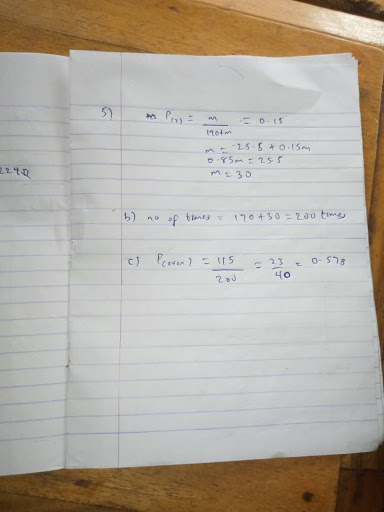2020/2021 WAEC General mathematics Obj and Essay : TO SUBSCRIBE FOR_2020WAEC MATHEMATICS EXPO FOLLOW THE LINKS BELOW .WAEC 2020/2021 mathematics Essay /Theory and Obj) Question and answers

### How to subscribe for WAEC 2020/2021 General mathematics Essay /Theory and Obj) Question and answers

2020 GENERAL MATHEMATICS  VIP

General Mathematics/Mathematics (Core) 1 (Objective)*
_3:00 pm – 4:30 pm_

Mathematics Obj is out....Add caption

(1a)
Given A={2,4,6,8,...}
B={3,6,9,12,...}
C={1,2,3,6}
U= {1,2,3,4,5,6,7,8,9,10}

A' = {1,3,5,7,9}
B' = {1,2,4,5,7,8,10}
C' = {4,5,7,8,9,10}
A'nB'nC' = {5, 7}

(1b)
Cost of each premiere ticket = \$18.50
At bulk purchase, cost of each = \$80.00/50 = \$16.00

Amount saved = \$18.50 - \$16.00
=\$2.50

=================

(2ai)
P = (rk/Q - ms)⅔
P^3/2 = rk/Q - ms
rk/Q = P^3/2 + ms
Q= rk/P^3/2 + ms

(2aii)
When P =3, m=15, s=0.2, k=4 and r=10
Q = rk/p^3/2 + ms = 10(4)/(3)^3/2 + (15)(0.2)
= 40/8.196 = 4.88(1dp)

(2b)
x + 2y/5 = x - 2y
Divide both sides by y
X/y + 2/5 = x/y - 2
Cross multiply
5(x/y) - 10 = x/y + 2
5(x/y) - x/y = 2 + 10
4x/y = 12
X/y = 3
X : y = 3 : 1

=====================
(3a)
Diagram
CBD = CDB (base angles an scales D)
BCD+CBD+CDB=180° (Sum of < in a D)
2CDB+BCD=180°
2CDB+108°=180°
2CDB=180°-108°=72°
CDB=72/2=36°
BDE=90°(Angle in semi circle)
CDE=CDB+BDE
=36°+90
=126

(3b)
(Cosx)² - Sinx given
(Sinx)² + Cosx
Using Pythagoras theory thrid side of triangle
y²= 1²+√3
y²= 1+ 3=4
y=√4=2
(Cosx)² - sinx/(sinx)² + cosx

(1/2)² - √3/2/
(√3/2)² + 1/2 = 1/4 - √3/2 = 1-2√3/4
3/4+1/2 = 3+2/4
=1-2√3/4 * 4/5
=1-2√3/5

=====================

(4a)
Total Surface Area = 224πcm²
r:l = 2:5
r/l = 2/5
Cross multiply
2l/2 = 5r/2
L = 5r / 2
Total surface = πrl + πr²
= πr (l + r)
24π/π = πr (5r/2 + r )/ π
224 = 5r²/2 + r²/1
L.c.m = 2

448 = 5r² + 2r²

448 / 7= 7r²/7

r² = 64
r = √64 = 8cn
L = 5*8/2 = 20cm

(4b)
Volume = 1/2πr²h
= 1/3 * 22/7 * 8 * 8 * 18.33
= 1228.98cm³
L² = h² + r ²
20² = h² + 8²
400 - 64 = h²
h² = 336
h = √ 336
h = 18.33cm

=======================

(5a)
Total income = 32+m+25+40+28+45
=170+m
PR(²)=m/170+m = 0.15/1
M=0.15(170+m)
M=25.5+0.15m
0.85m/0.85=25.5/0.85
M=30

(5b)
Total outcome = 170 + 30 = 200

(5c)
PR(even numbers) = 30+40+50/200
=115/200 = 23/40

==========================

=== SECTION B ====
================================

Using Pythagoras theorem, l²=48² + 14²
l²=2304 + 196
l²=2500
l=√2500
l=50m
Area of Cone(Curved) =πrl
Area of hemisphere=2πr²
Total area of structure =πrl + 2πr²
=πr(l + 2r)
=22/7 * 14 [50 + 2(14)]
=22/7 * 14 * 78
=3432cm²
~3430cm² (3 S.F)

(b)
let the percentage of Musa be x
Let the percentage of sesay be y
x + y=100 -------------------1
(x - 5)=2(y - 5)
x - 5=2y - 10
x - 2y=-5 -------------------2

Equ (1) minus equ (2)
y - (-2y)=100 - (-5)
3y=105
y=105/3
y=35
Sesay's present age is 35years

====================================
(8a)
Let Ms Maureen's Income = Nx
1/4x = shopping mall
1/3x = at an open market

Hence shopping mall and open market = 1/4x + 1/3x
= 3x + 4x/12 = 7/12x

Hence the remaining amount
= X-7/12x = 12x-7x/12 =5x/12

Then 2/5(5x/12) = mechanic workshop
= 2x/12 = x/6
Amount left = N225,000
Total expenses
= 7/12x + X/6 + 225000
= Nx

7x+2x+2,700,000/12 =Nx
9x + 2,700,000 = 12x
2,700,000 = 12x - 9x
2,700,000/3 = 3x/3
X = N900,000

(ii) Amount spent on open market = 1/3X
= 1/3 × 900,000
= N300,000

(8b)
T3 = a + 2d = 4m - 2n
T9 = a + 8d = 2m - 8n
-6d = 4m - 2m - 2n + 8n
-6d = 2m + 6n
-6d/-6 = 2m+6n/-6
d = -m/3 - n
d = -1/3m - n

====================================

(9a)
Draw the triangle

(9b)
(i)Using cosine formulae
q² = x² + y² - 2xycosQ
q² = 9² + 5² - 2×9×5cos90°
q² = 81 + 25 - 90 × 0
q² = 106
q = square root 106
q = 10.30 = 10km/h
Distance = 10 × 2 = 20km

(ii)
Using sine formula
y/sin Y = q/sin Q
5/sin Y = 10.30/sin 90°
Sin Y = 5 × sin90°/10.30
Sin Y = 5 × 1/10.30
Sin Y = 0.4854
Y = sin‐¹(0.4854), Y = 29.04

Bearing of cyclist X from y
= 90° + 19.96°
= 109.96° = 110°

(9c)
Speed = 20/4, average speed = 5km/h

(7a)
Total surface area = url + 2πr²
=πr(l + 2r)

Draw the diagram
From pythagoras theorem
L² = 14² + 48²
L² = 196 + 2304
L² = 2500
L = /2500 = 50m

=πr(L + 2r)
= 22/7 ×14(50 + 2(14))
= 44(50 + 28)
= 3432m²
Total surface area = 3432m²
~3430m²(to 3s.f)

(7b)
Five years ago,
Let Musa's age = x
Let Sesay's age = y
X - 5 = 2(Y - 5)
X - 5 = 2y - 10
X - 2y = 5 - 10
X - 2y = -5 ..... (1)
-X + y = 100 ..... (2)
-3y = -105
Subtracting eqn 2 from 1
-3y/3 = -105/-3
y = 35
Sesay's present age = 35 years

(12a)
BCD=ABC=40°(alternate D)

DDE=2*BCD(<at centre = twice < at circle)

DDE = 2*40 = 80°
OD3=OED(base < of I sealed D ODE)
ODE + OED + DOE= 180°(sum of < is in D)
2ODE+DOE=180°
2ODE+80°=180
2ODE+180=180
2ODE+100°
ODE+100/2=50°

(12bi)
Digram

(12bii)
Area of parallelogram = absin
=5*7*sin125°
=35*sin55°
=35*0.8192
=28.67
=28.7cm²(1dp)

(12c)
Given x=1/2(1-√2)
2x²-2x=2[1/2(1-√2]²-2(1/2(1-√2)}
=2[1-2√2+2/4]-(1-√2)
=(3-2√2/2)-(1-√2)
=3-2√2-2+2√2/2=1/2

KEEEP REFRESHING THE PAGES......